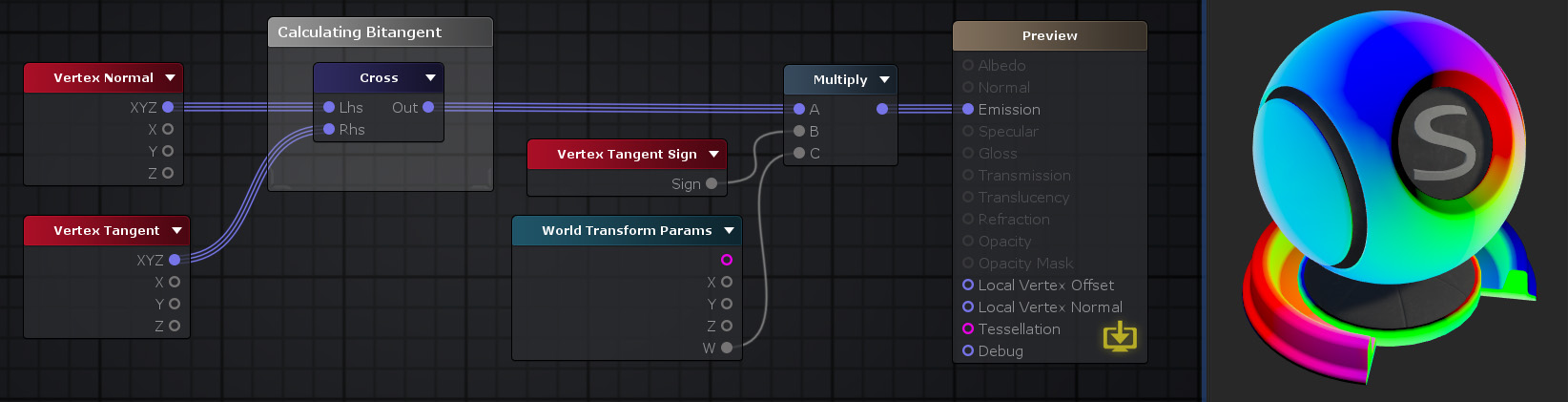## Cross Node

The Cross node ( shortcut: X key ) calculates a cross/vector product between two Vectors A and B. Cross product is a operation between two three dimensional/Float3 vectors which generates another vector.
If both A and B vectors are linearly independent between each other then the resulting vector will be perpendicular to both of them and will represent a normal vector for the plane they produce.

NOTE: Order is important on this operator as AxB outputs different results than BxA ( which result is similar to -AxB ).Nodes used: Vertex Normal, Vertex Tangent, Vertex Tangent Sign, World Transform Params, Multiply

Node Parameter Description Default Value
Lhs First, or left hand side, value of the cross product. Only visible if the respective input port is not connected. 0,0,0
Rhs Last, or right hand side, value of the cross product. Only visible if the respective input port is not connected. 0,0,0

Input Port Description Type
Lhs First, or left hand side, value of the cross product. Float3
Rhs Last, or right hand side, value of the cross product. Float3